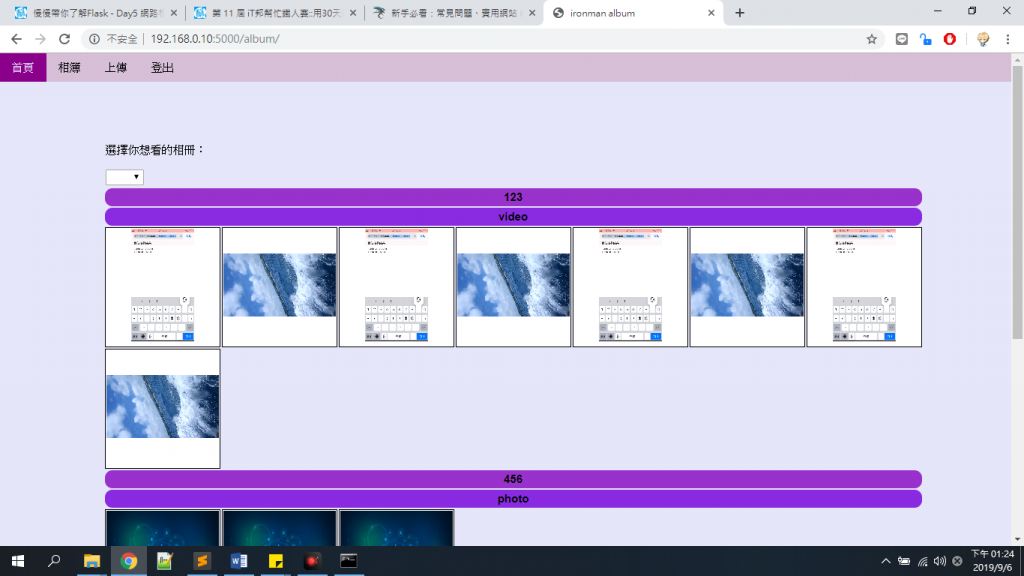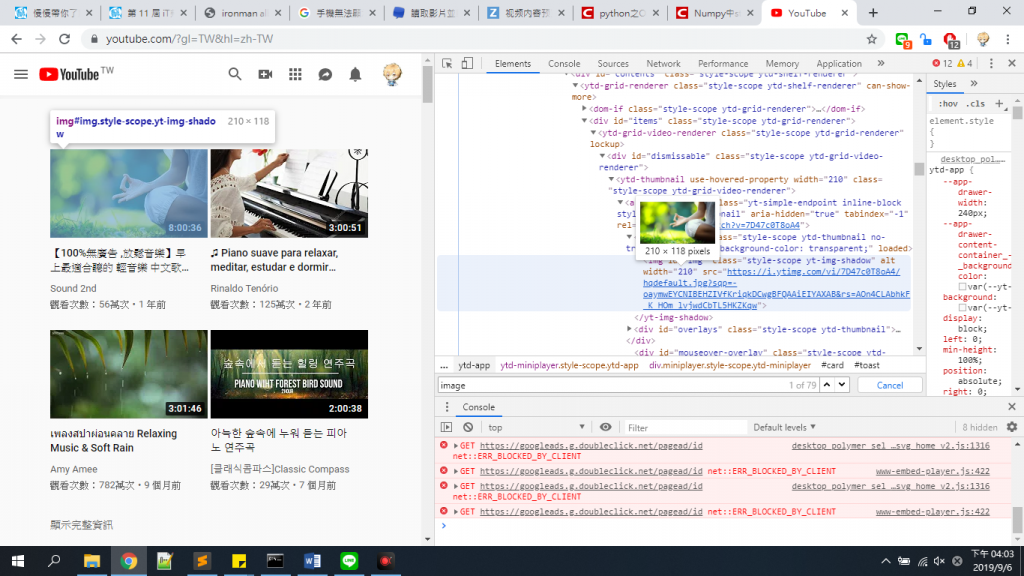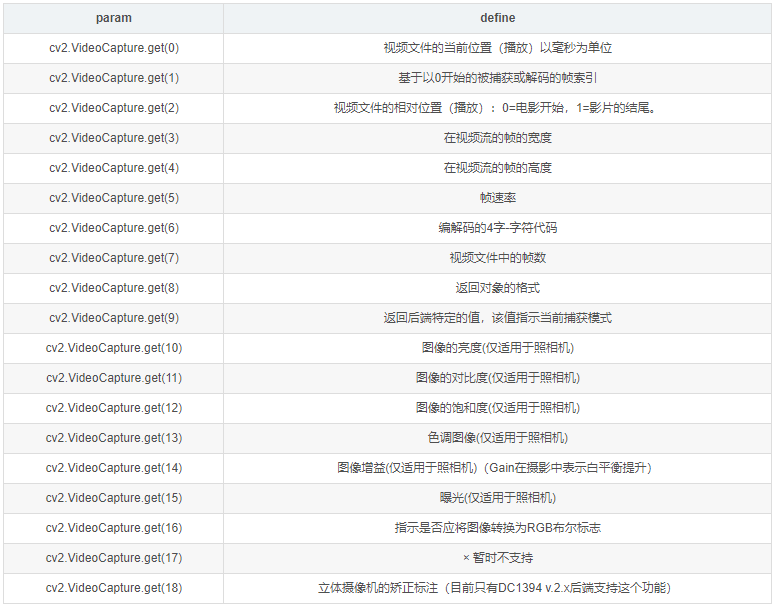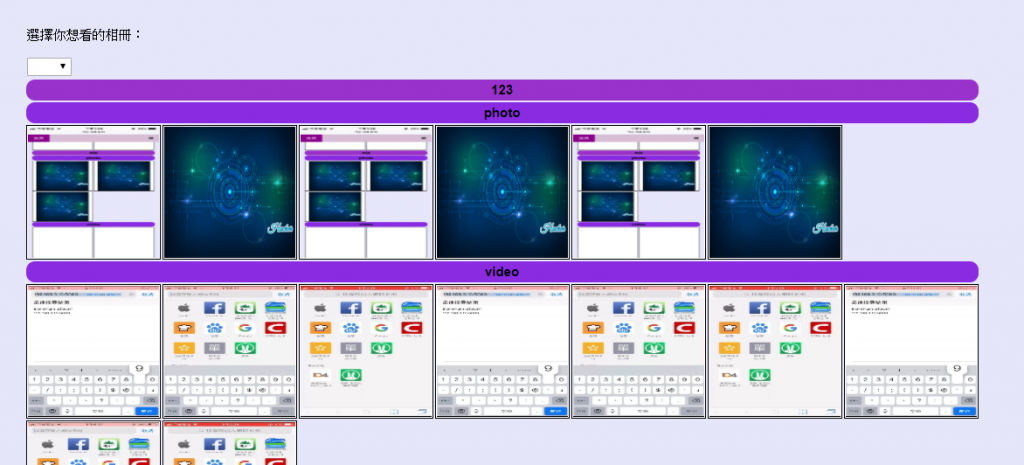#DAY 9
3
Modern Web

(看不懂我說什麼沒關係，我自己也覺得我講的很奇怪，直接看實作後吧！)https://github.com/zhaoxuhui/VideoPreviewer

``````import cv2
import numpy as np

video_path = input("Input path of video:\n")
max_width=150
out_path = 'content.jpg'

cap = cv2.VideoCapture(video_path)
video_width = int(cap.get(3))

ratio = max_width * 1.0 / video_width

image=cv2.resize(frame, None, fx=ratio, fy=ratio)
cap.release()

cv2.imwrite(out_path, image)
``````

`pip install numpy` numpy 通常是處理資料組
`pip install opencv-python`這是個可以處理圖片的module

`cv2.VideoCapture(video_path)`是讀取影片檔案`cap.read()`會回傳兩個參數，第一個是是否讀到圖片(True跟False)，第二個則是截取一幀圖片。
`resize`字面意思，將圖片依比率放大縮小。
`cap.release()`既然我們都得到我們要的image了，那我們就應該釋放資源。
`cv2.imwrite(out_path, image)`將我們的image存在設定好的路徑

`import cv2`
`import numpy as np`

``````				elif request.values['folder']=='1':
``````

``````if format == '.mp4':

else:
else:
if format == '.mp4':
else:

``````

``````				if format =='.mp4':
``````

``````def video_photo(video_path,out_path):
max_width=150

cap = cv2.VideoCapture(video_path)
video_width = int(cap.get(3))

ratio = max_width * 1.0 / video_width

image=cv2.resize(frame, None, fx=ratio, fy=ratio)
cap.release()

cv2.imwrite(out_path, image)
``````Wow!!!已經呈現我們想像中的樣子啦！### 1 則留言

1
``````有沒有設定說 ，如果我丟一個`不公開的相片`或影片的 權限方面調整 ?
``````